# How to Create Contour Lines in QGIS

This tutorial will discuss how to create contour lines in QGIS. But before we are discussing about drawing contour lines in QGIS, let's start talking a little bit about the concept of contour line, what contour line definition is, contour lines characteristics and and how the method in drawing contour line . In the last section we will see how to create contour lines from points and also from DEM in QGIS. For this tutorial I am using QGIS 3.0.

### What is Contour Line?

Contour is a height isoline. Then what is an isoline? Isoline is a line on a map with a constant value. Therefore the contour definition is a line which has a constant elevation along the line. There are some characteristics of contour line such as contour interval, contour elevation and distance between contour lines. If we see a contour map we found many lines on it. Those lines are never crossing to each other and each line has a unique elevation value that define its elevation on the map. Contour interval defines height interval between the line. For example if a contour map has 5 m interval contour, it means the height different between the adjacent contour line is 5 m. If we know the distance between contour lines then we can calculate the slope. The rule of thumb in defining contour interval is 1/2000 x map scale.

### How to Draw Contour Lines

The basic method in drawing contours line is interpolation from a point dataset. The simplest interpolation method is linear interpolation which is frequently used in drawing contours lines manually.  In drawing a contour line with linear interpolation, at least three steps need to be done:
1. Connecting each point to form a TIN (Triangular Irregular Network) structure.
2. Do interpolation for each line in the TIN.
3. Connecting the interpolation point with the same elevation.
Figure 1 shows step by step approach in drawing contour line from connecting each point to form TIN, do interpolation along the TIN line and drawing contour line by connecting the same elevation of interpolation points.Figure 1. Contour line drawing
We already got some basic concepts about contour line. Now let's move to technical part how to create contour lines with QGIS. Firstly we will see how to create contour line from a set of height points.

### Create Contours from Points

To create contour lines from points, we are using Contour plugin. As usual we can get the plugin from Plugins menu >> Manage and install plugins. In the search option type "Contour". You will see the Contour plugin appears as in figure 2. Check it and Install the plugin if you don't have it in your QGIS 3.Figure 2. Installing Contour Plugin
Now, let's make contour lines from points dataset with the following steps:

1. Add the points into QGIS map canvas as in figure3.Figure 3. Points dataset
2. Open the Contour plugin by clicking the icon. The contour plugin window will appear as in figure 4.
3. Select the point's layer name and the elevation data field. In the contouring menu, we can choose  to create contour line, filled contour or both. Next, we have to define the number of contour lines. We can set any number, but I suggest the number of contour line follows the rule of thumb as mentioned above. For example if we want to make a contour map in 100.000 scale, then the contour interval will be 1/2000 x 100.000 = 50 m. Then the number of contour line will be: maximum elevation/contour interval. 3554/50=71 lines. When we set the calculated number, the contour interval can be seen in the right list. We can tweak the number if the interval is not correct. That's why I change the number to 72. Moreover the interval can be edited manually by double click the interval number.
4. Next we can specify the output name. The output will be written to memory, so we need to export to it to a GIS file to keep it permanently.
5. In the next setting we can specify the precision and unit for the contour label. Furthermore the color graduation for the contour lines can be changed by selecting a color ramp for the output contour lines.
6. When finish click the Add button. The result can be seen in the QGIS map canvas without closing the contour plugin window, therefore we can switch back to change a parameter if the result not looks as we want. Figure 5 shows the result of contour lines.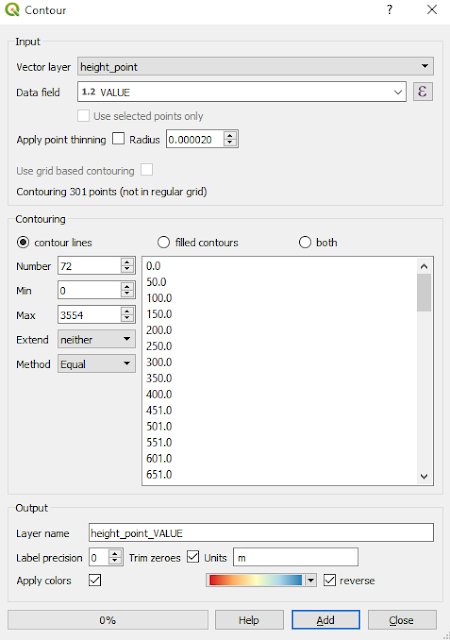Figure 4. Contour plugin windowFigure 5. Contour lines result from points

### Create Contours from DEM

In this part we will create contour lines from DEM. To create contour lines from DEM we can use the Contour tool in the Raster menu >> Extraction >> Contour. See figure 6.Figure 6. Contour tool
To create the contour lines from DEM, can be done with several steps:
1. Add DEM data into QGIS map canvas, as in figure 7. If you don't have one. You can download DEM data directly from QGIS.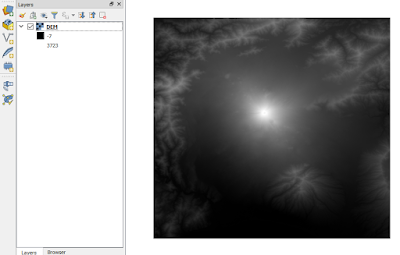Figure 7. DEM data in QGIS map canvas
2. Open the Contour tool. The contour tool window will appear as in figure 8.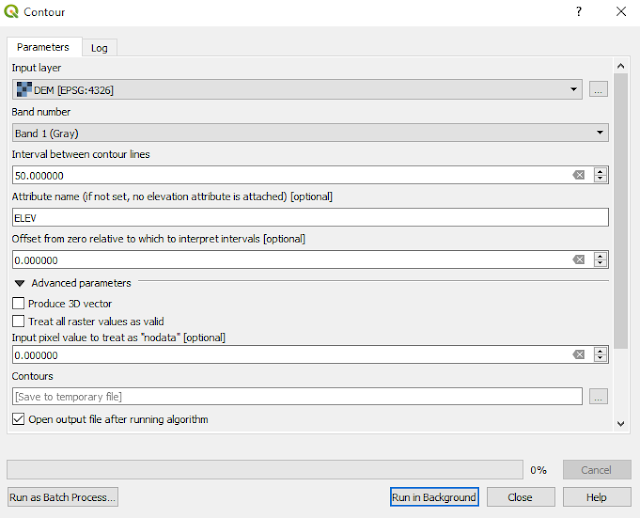Figure 8. Raster contour tool
3. Select the DEM data. Then set the contour interval, in this case  I used 50 m. In the Contours option, we can specify the contour output path or save it to temporary file.
4. If all the settings already set. Click the Run in Background button. The result will shown in QGIS  map canvas as in figure 9.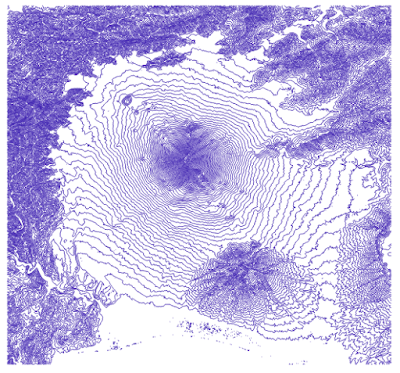Figure 9. Contour lines from DEM

### Contour Smoothing

If we compare the result, the contour lines from DEM looks smoother than points. That's because the DEM contains more elevation information compare to points dataset. The DEM has information in each pixel, so it could consist of thousands pixel and elevation information. But don't worry. We can make the contour line from points smoother using Smooth tool as follow:

1.  In the processing toolbox type "smooth". The smooth tool will appear under  Vector Geometry, as in figure 10.Figure 10. Vector geometry smooth tool
2. Open the smooth tool. Select the input contour layer. Set the number of iteration, offset and maximum angle to smooth. The explanation of each parameter can be seen in the help window on the right. See figure 11.Figure 11. Contour smoothing
3. If all the parameters have been set. Click Run in Background button to start the smoothing process. Figure 12 shows the comparison of contour lines before and after smoothing.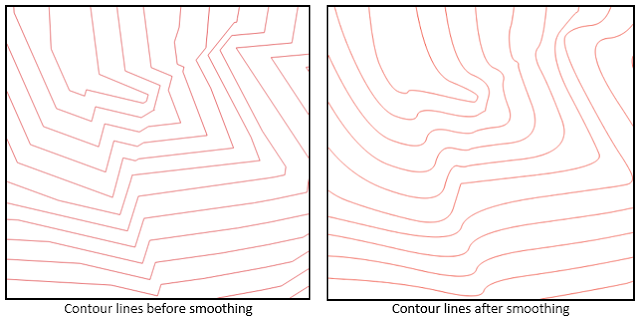Figure 12. Contour lines before and after smoothing

That's all the tutorial how to create contour lines in QGIS both from points and DEM. I hope it's useful. Please give comment if you find another tool in QGIS or any improvement in creating contour lines using QGIS software. Anyway, in creating contour lines some tools are used as already explained. It's better to build a model for it which can automate all the tools and can be used repeatedly. Check out this tutorial how to create a model builder in QGIS.